In :
# %load /Users/facai/Study/book_notes/preconfig.py
%matplotlib inline

import matplotlib.pyplot as plt
import seaborn as sns
sns.set(color_codes=True)
sns.set(font='SimHei', font_scale=2.5)
plt.rcParams['axes.grid'] = False

#import numpy as np

#import pandas as pd
#pd.options.display.max_rows = 20

#import sklearn

#import itertools

#import logging
#logger = logging.getLogger()

#from IPython.display import SVG
def show_image(filename, figsize=None, res_dir=True):
if figsize:
plt.figure(figsize=figsize)

if res_dir:
filename = './res/{}'.format(filename)



# 10 Sequence Modeling: Recurrent and Recursive Nets¶

RNN: to process sequential data

traditionaly fully connected feedforward network: separate parameters at each position. => recurrent neural network: shares the same parameters across several steps.

### 10.1 Unfoliding Computational Graphs¶

recurrent neural networks: $h^{(t)} = f(h^{(t-1)}, x^{(t)}; \theta)$

In :
show_image('fig10_2.png', figsize=(12, 5))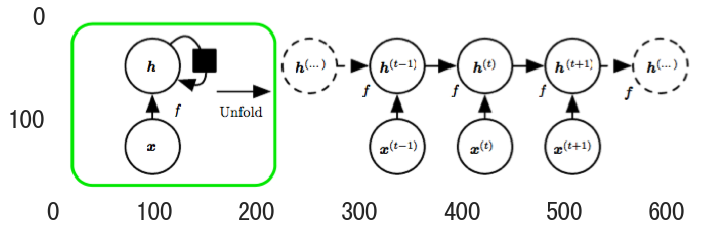• same input size even for a variable-length sequence.
• use the same transition function $f$ with the same parameters at every time step.

### 10.2 Recurrent Neural Networks¶

Different recurrent networks:

In :
# A.
show_image('fig10_3.png', figsize=(10, 8))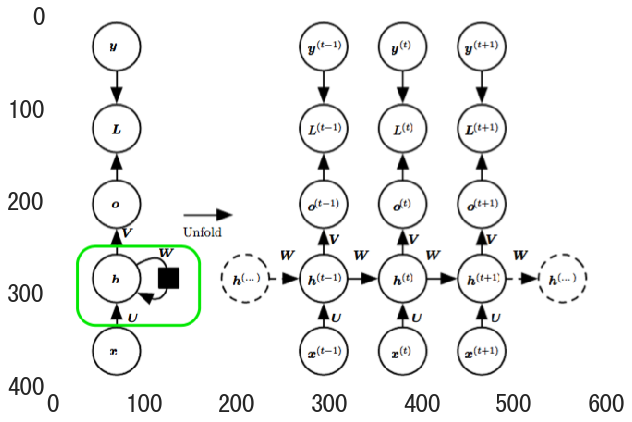\begin{align} a^t &= b + W h^{(t-1)} + U x^t \\ h^t &= \tanh(a^t) \\ o^t &= c + V h^t \\ \hat{y}^t &= \operatorname{softmax}(o^t) \\ \end{align}

The total loss for a given sequence of $x$ values paired with a sequence of $y$ values would be just the sum of the losses over all the time steps:

\begin{align} &L \left ( \{x^1, \cdots, x^\tau\}, \{y^1, \cdots, y^\tau\}) \right ) \\ &= \sum_t L^t \\ &= - \sum_t \log p_{\text{model}} \left ( y^t \, | \, \{x^1, \cdots, x^\tau\} \right ) \\ \end{align}

So the back-propagation algorithm need $O(\tau)$ running time moving right to left through the graph, and also $O(\tau)$ memory cost to store the intermediate states. => back-propagation through time (BPTT): powerful but also expensive to train

In :
# B.
show_image('fig10_4.png', figsize=(10, 8))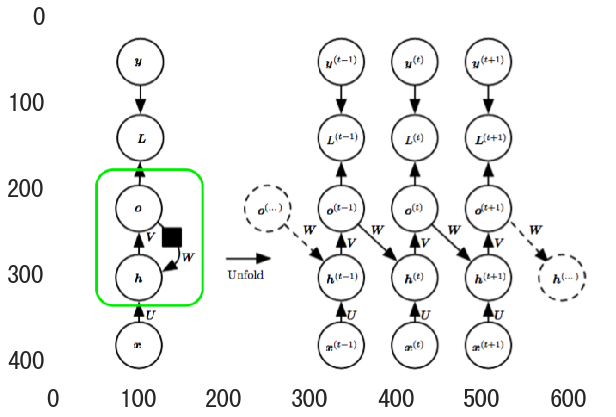advantage of eliminating hidden-to-hidden recurrence: decouple all the time steps (replace predition by its ground truth in sample for $t-1$) => teacher forcing

In :
show_image('fig10_6.png', figsize=(10, 8))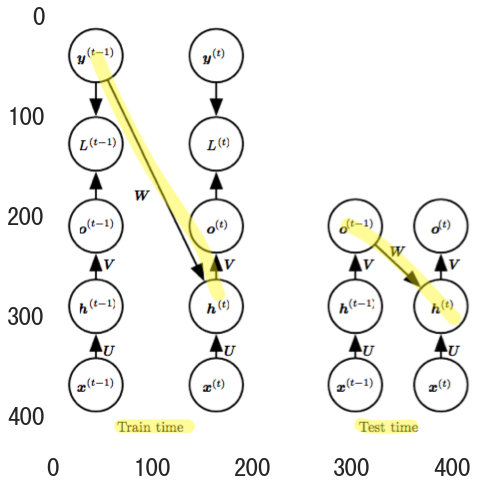disadvantage: open-loop network (outputs fed back as input) => inputs are quite different between training and testing.

• mitigate the problem:
1. train with both teacher-forced inputs and with free-running inputs.
2. randomly choose to use generated values or actual data as input.
In :
# C.
show_image('fig10_5.png', figsize=(10, 8))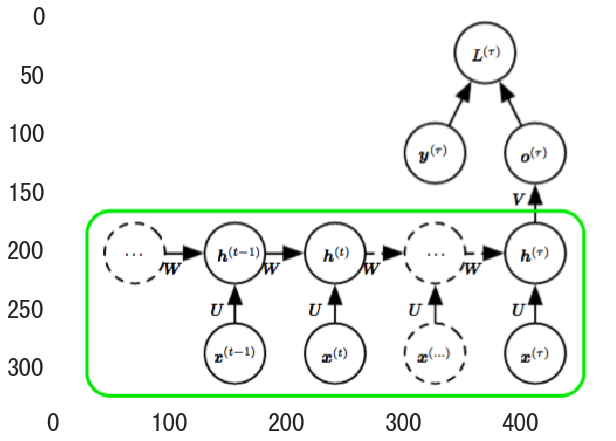#### 10.2.2 Computing the Gradient in a Recurrent Neural Network¶

1. calculate the gradients on the internal nodes.
2. calculate the gradients on the paramter nodes. Because the parameters are shared across many time steps, we introduce dummy variables $W^t$ that are defined to be copies of $W$ but with each $W^t$ used only at time step $t$.
In :
show_image("formula_gradient.png", figsize=(12, 8))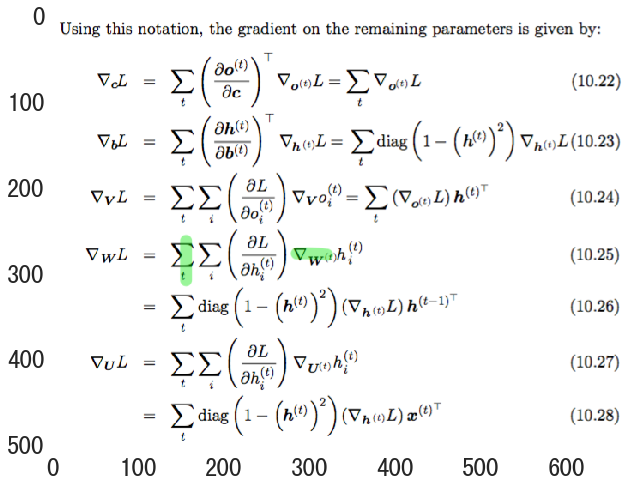#### 10.2.3 Recurrent Networks as Directed Graphical Models¶

Mean squared error: the cross-entropy loss associated with an output distribution that is a unit Gaussian.

In :
show_image('fig10_7.png', figsize=(10, 8))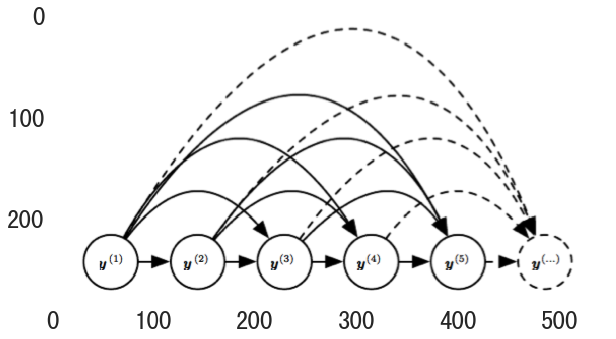RNNs obtain the same full connectivity above but efficient parametrization, as illustrated below:

• Incorporating the $h^t$ nodes in the graphical model decouples the past and the future.
• Variable $y^i$ in the distant past may influence $y^t$ via its effect on $h$.
• Assume same conditional probability distributions at each time step. $\gets$ parameter sharing
In :
show_image('fig10_8.png', figsize=(10, 8))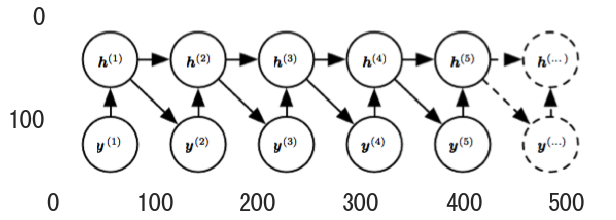determining the length of the sequence:

• add a special symol in training sample.
• introduce an extra Bernoulli output: decision to either continue generation or halt at each time step.
• add an extra output to predict length $\tau$.

#### 10.2.4 Modeling Sequences Conditioned on Context with RNNs¶

In :
# fixed-length vector x, share R
show_image('fig10_9.png', figsize=(10, 8))